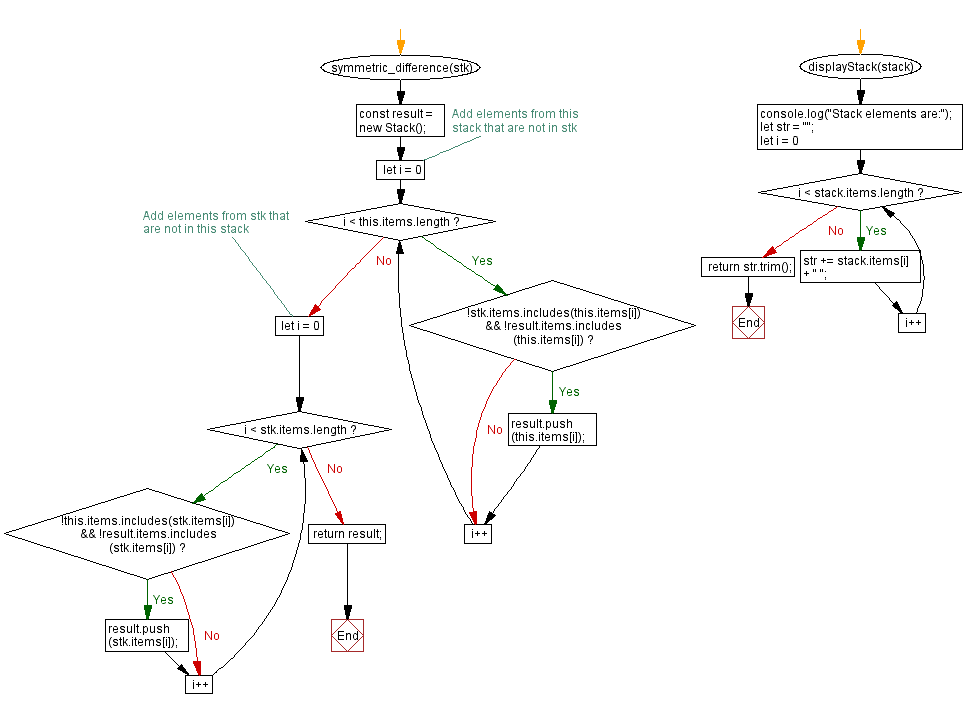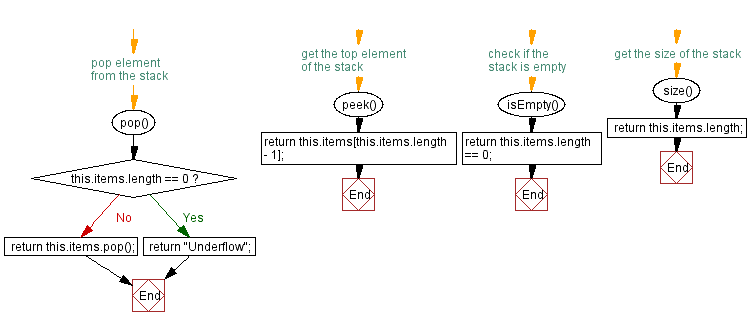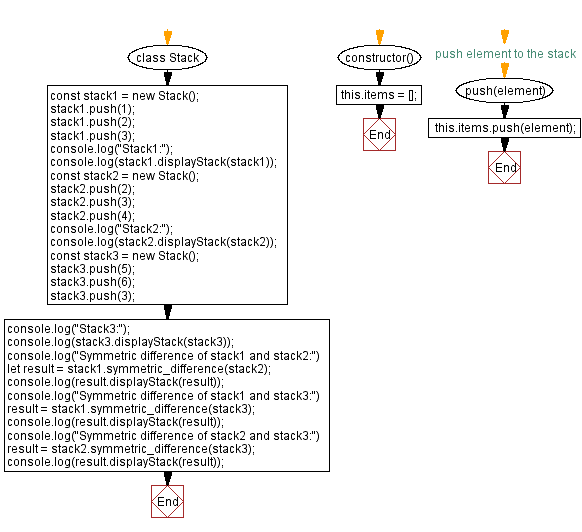# JavaScript Exercises: Symmetric difference of two stacks

## JavaScript Stack: Exercise-31 with Solution

Write a JavaScript program that implements a stack and creates a new stack that contains only elements that are in either the first or the second stack, but not in both.

Sample Solution:

JavaScript Code:

``````class Stack {
constructor() {
this.items = [];
}

// push element to the stack
push(element) {
this.items.push(element);
}

// pop element from the stack
pop() {
if (this.items.length == 0) {
return "Underflow";
}
return this.items.pop();
}

// get the top element of the stack
peek() {
return this.items[this.items.length - 1];
}

// check if the stack is empty
isEmpty() {
return this.items.length == 0;
}
// get the size of the stack
size() {
return this.items.length;
}
symmetric_difference(stk) {
const result = new Stack();

// Add elements from this stack that are not in stk
for (let i = 0; i < this.items.length; i++) {
if (!stk.items.includes(this.items[i]) && !result.items.includes(this.items[i])) {
result.push(this.items[i]);
}
}

// Add elements from stk that are not in this stack
for (let i = 0; i < stk.items.length; i++) {
if (!this.items.includes(stk.items[i]) && !result.items.includes(stk.items[i])) {
result.push(stk.items[i]);
}
}

return result;
}

displayStack(stack) {
console.log("Stack elements are:");
let str = "";
for (let i = 0; i < stack.items.length; i++)
str += stack.items[i] + " ";
return str.trim();
}
}

const stack1 = new Stack();
stack1.push(1);
stack1.push(2);
stack1.push(3);
console.log("Stack1:");
console.log(stack1.displayStack(stack1));
const stack2 = new Stack();
stack2.push(2);
stack2.push(3);
stack2.push(4);
console.log("Stack2:");
console.log(stack2.displayStack(stack2));
const stack3 = new Stack();
stack3.push(5);
stack3.push(6);
stack3.push(3);
console.log("Stack3:");
console.log(stack3.displayStack(stack3));
console.log("Symmetric difference of stack1 and stack2:")
let result = stack1.symmetric_difference(stack2);
console.log(result.displayStack(result));
console.log("Symmetric difference of stack1 and stack3:")
result = stack1.symmetric_difference(stack3);
console.log(result.displayStack(result));
console.log("Symmetric difference of stack2 and stack3:")
result = stack2.symmetric_difference(stack3);
console.log(result.displayStack(result));
```
```

Sample Output:

```Stack1:
Stack elements are:
1 2 3
Stack2:
Stack elements are:
2 3 4
Stack3:
Stack elements are:
5 6 3
Symmetric difference of stack1 and stack2:
Stack elements are:
1 4
Symmetric difference of stack1 and stack3:
Stack elements are:
1 2 5 6
Symmetric difference of stack2 and stack3:
Stack elements are:
2 4 5 6
```

Flowchart:Live Demo:

See the Pen javascript-stack-exercise-31 by w3resource (@w3resource) on CodePen.

Improve this sample solution and post your code through Disqus

Stack Previous: Elements from both stacks without duplicates.
Stack Exercises Next: Extract a portion from the said stack.

What is the difficulty level of this exercise?

Test your Programming skills with w3resource's quiz.

﻿